# Modulation Recognition Using Cyclic Cumulants, Part I: Problem Description and Variants

In this post, we start a discussion of what I consider the ultimate application of the theory of cyclostationary signals: Automatic Modulation Recognition. My relevant papers are My Papers [16,17,25,26,28,30,32,33,38,43,44].

### What is Modulation Recognition?

Modulation Recognition (MR) is the ability to assign a modulation-type label to a captured RF signal. A “modulation-type label” just means the basic modulation scheme associated with the RF signal, such as binary phase-shift keying (BPSK), Gaussian Minimum-Shift Keying (GMSK), amplitude modulation (AM), etc.  Automatic modulation recognition (AMR) is the ability of a computer system to accurately assign a modulation-type label to an RF signal without human assistance. AMR is also referred to as automatic signal classification, or just signal classification.

Modulation recognition can be used as a prelude to communication-signal demodulation when the exact modulation type of the transmitted signal is unknown to the receiver. This can happen in adaptive radio, where the transmitter may make a change to the modulation type, or in intelligence and surveillance settings, where the transmitted message may have high value. MR is also applicable to general spectrum monitoring for, say, purposes of frequency-assignment enforcement or to identify malfunctioning or rogue radios. It can also be used as a prelude to communication network set up and frequency assignments; what signals are present and are there any suitable spectrum holes or underlay/overlay opportunities?

### Major Modulation Recognition Problem Variants

I’ll start with the most general variant, which I call radio-frequency scene analysis (RFSA), because it is the most challenging and, arguably, the most interesting to a general audience. Here one is confronted with a wideband scenario and the problem is to identify and characterize each and every signal present in the scenario, including any that might be hiding below the noise floor, or hiding in the same frequency band as another signal. By identify I mean detect and assign a modulation-type label. By characterize I mean estimate all relevant parameters for the signal–which may depend on the assigned signal-type label–such as symbol rate, carrier frequency, chip rate, hop rate, pulse-shaping function, excess bandwidth, processing gain, etc. For example, here is a captured scenario (absolute carrier frequency set to zero and original sampling rate set to unity here):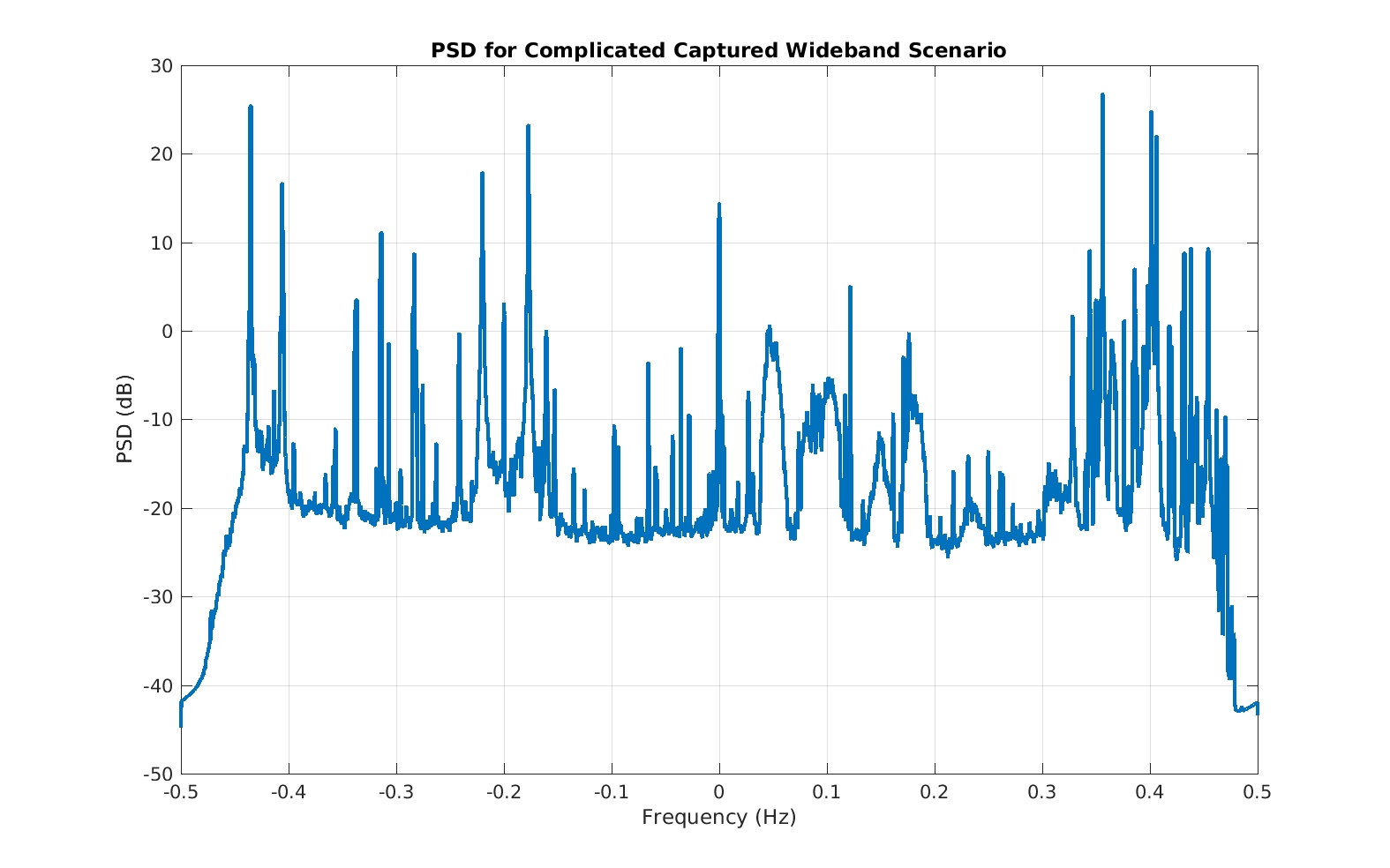A simulated version of such a complicated scenario is show next: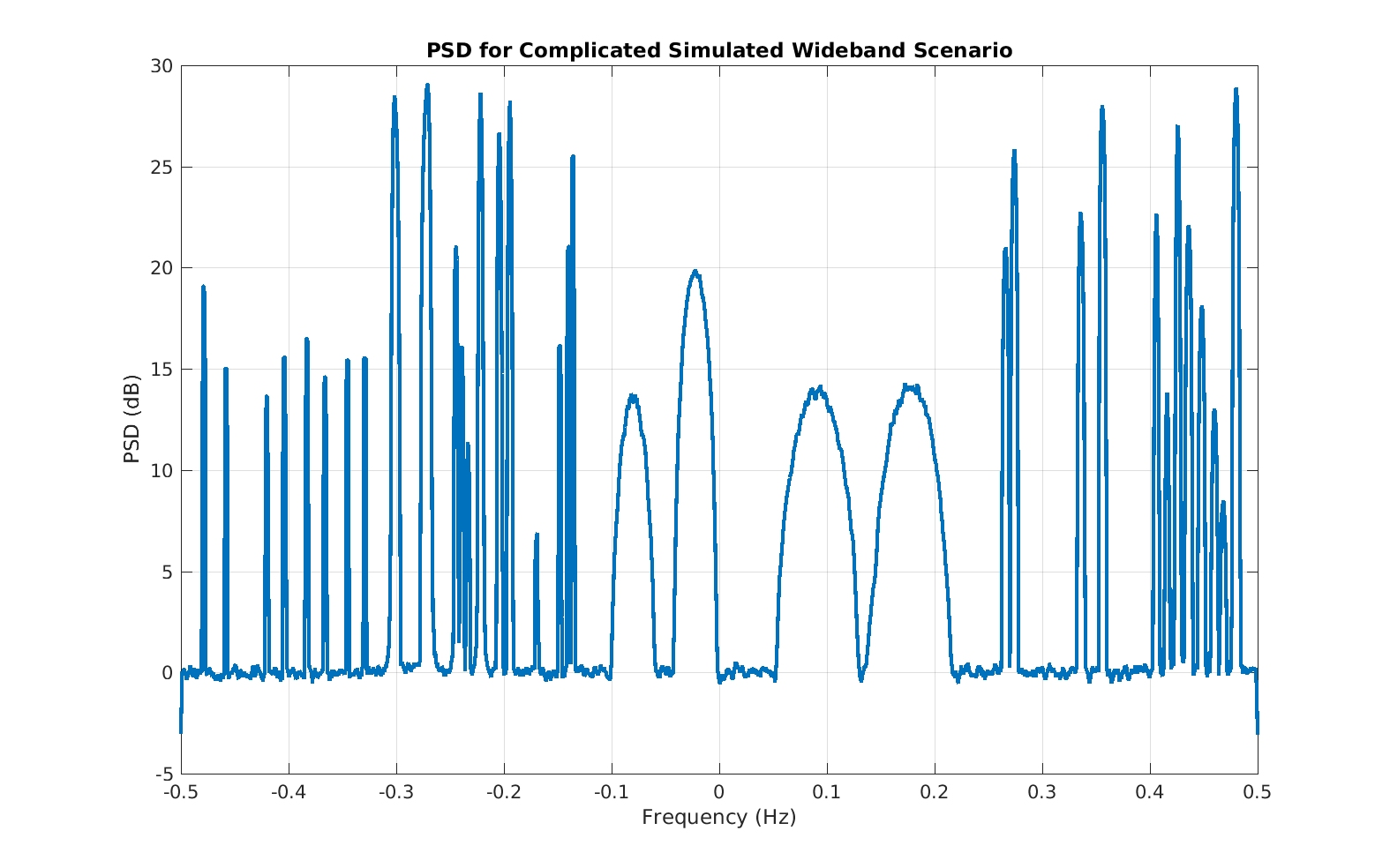Don’t let the apparent high-SNR of most of the signals fool you: many of the bumps in that PSD contain two or more highly overlapped cochannel signals!

One thing that is immediately clear in the RFSA problem is that the provided data must somehow be segmented in frequency. We’ll cover my method for doing this in a future post (see My Papers ). In other words, it would be advantageous to first isolate (without imparting significant distortion) each occupied subband in the scene. Then we can process the subbands’ data sequentially. Automatic blind spectral segmentation is a hard problem in its own right. It is complicated in practice by the presence of non-flat and/or time-varying noise floors and adjacent-channel signals that are not well isolated in frequency.

The second modulation classification variant we’ll call conventional modulation classification (CMC). This problem variant features a small catalog of signal types to choose from, and often significant prior information such as symbol rate and carrier-offset frequency. For example, the classifier is provided the complex envelope data for a captured digital QAM signal and must decide between, say, four constellations: BPSK, QPSK, 16QAM, and 64QAM. So the CMC problem is often a problem of constellation identification or estimation. Most of the papers on MR in the literature correspond to the CMC problem variant.

The third variant is the cognitive-radio modulation classification (CRMC) problem. Recall that in cognitive radio, the secondary users are permitted to transmit on a frequency band that is owned by some primary user whenever that primary user is not using the band. So the secondary users periodically sense the appropriate subbands in an attempt to detect the presence of the primary user’s signal. It has overwhelmingly been proposed to perform this function using energy detection (My Papers ). If the detector raises an alarm, how are we to know if the detected signal energy is really from the primary or not? It could be simply spectrally shaped noise, as in a primary-user emulation attack. One step toward verifying the authenticity of the signal is by performing modulation recognition. Thinking of IEEE 802.22, is there an ATSC digital TV signal in this figure, just a noisy tone, or a tone plus spectrally shaped noise:The fourth variant includes all manner of scenarios involving multiple spectrally overlapping signals. We’ll call it the cochannel modulation classification (CCMC) variant. In traditional RF settings and in mathematically friendly models, signals are not piled on top of each other in a frequency channel. There are situations in which cochannel signals can be observed, and even some situation in which the cochannel signals are intentionally transmitted together. In most cases I know of, however, it is the signal collection geometry together with the practice of terrestrial-system frequency-reuse that gives rise to captured data containing cochannel signals. In other words, if you were on a mountaintop looking down into a broad valley, you might be equidistant (roughly) from multiple cellular/PCS systems that are reusing the same bands. From the point of view of the users on the surface of the Earth, the propagation from relatively distant cell towers causes cochannel signals to be very weak, but from the point of view of a far-off aerial or hill-top signal collector, the signals from multiple towers have comparable strengths, and may all be received by a suitably sensitive device.

In other situations, as I alluded to above, cochannel signals are a feature, not a bug.  Here is an illustration of multiple cochannel signals using simulated data: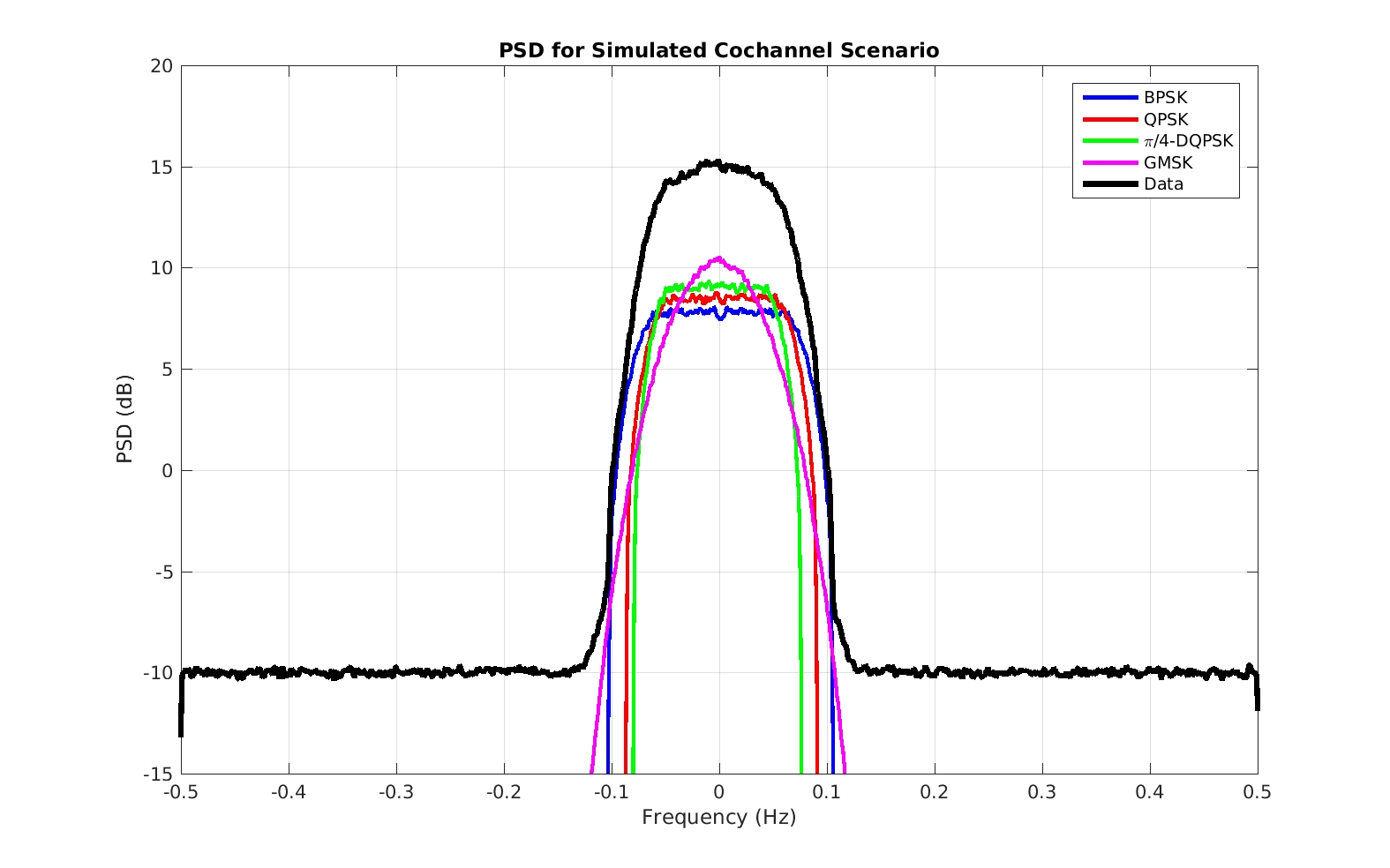This particular cochannel scenario is one that can be successfully analyzed with CSP, although it takes the careful application of several of our most complicated signal-processing tools to do it.

### Problem Dimensions

The many problem dimensions are what makes the modulation classification problem hard, but they also render it interesting and allow for several different development approaches. The mathematical approach one adopts depends heavily on the signal model implied by the problem context. Simple (and typically unrealistic) signal models lead to tractable optimal mathematical approaches, whereas more complicated (more realistic) signal models lead to suboptimal feature-driven approaches. A big barrier to achieving optimality is that the tractable simple models lead to solutions that are not easily extended to more complicated situations.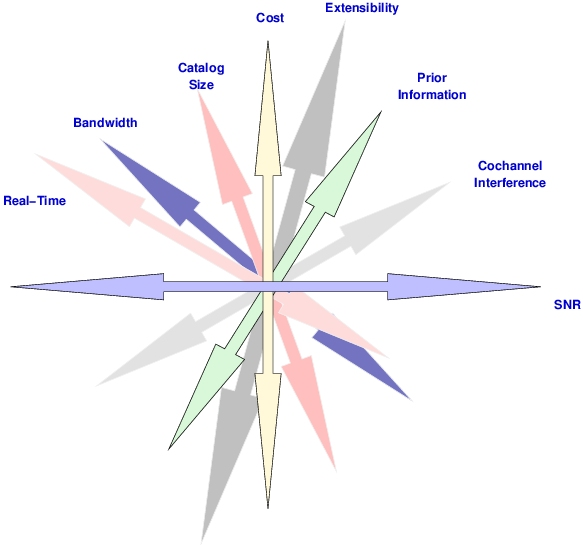Some problem dimensions are shown in the figure above. I’ve typically ignored computational cost and achievement of real-time processing in favor of accuracy and generality. The hope is a costly but accurate solution will be more attractive as time passes because computational power per unit cost continues to increase.

#### Catalog Size

The catalog size is the number of unique modulation types that can be distinguished by the classifier. In many papers in the literature, the catalog size is ten or less, and often it is equal to four. In RFSA, we want the catalog size to be much bigger; ideally it is equal to the number of actual modulation types in the world (how many is that I wonder?). In CMC the size is small by (my) definition, and in CRMC it is also quite small, being equal to the number of primary-user modulation types plus, optionally, the number of possible secondary-user modulation types.

Larger catalogs tend to discourage sophisticated mathematical treatments. This is because it is difficult to write a single equation that models all the modulation types in the catalog. That difficulty, in turn, hinders the application of decision theory (conventional hypothesis-testing mathematical machinery). Researchers that obsess over optimality then tend to pare the problem down to the point at which they can apply their favorite version of the machinery. So we end up with nice mathematical solutions to fake problems.

#### SNR

Noise is a vexing problem in modulation classification because the sensitivity of the decision-theoretic method or of the utilized features can be quite high. A relatively small amount of noise can ruin the discrimination power of some features, such as instantaneous frequency.

#### Cochannel Interference

Interference that resides in the same frequency band and time interval is commonly called cochannel interference. Like noise, cochannel interference can render some classification features useless, as these features reflect the presence of all the signals that reside in the band, not just the one you might care about. Unlike noise, cochannel interference can systematically modify a feature, rather than simply randomly degrade it, so that it looks very much like the feature would for some other high-SNR signal. This ends up fooling the classifier badly.

#### Prior Information

By “prior” we mean “prior to processing,” so that prior information is information relating to the signal, interference, or noise, that might be useful during either parameter/feature estimation or during the classification procedure itself. A simple example is knowledge of the symbol rate for a digital signal. Since the symbol rate is usually also a cycle frequency, this immediately leads to the possibility of a low-cost (no searches over cycle frequency) cycle detector. Other prior information could be the exact carrier frequency, the SNR, the pulse type (for applicable signal types of course), and a restriction on the catalog.

Prior information could be used to improve the performance of the algorithm, or it might be used to reduce computational costs (fewer searches over nuisance parameters, for instance), or both.

### Performance Measures for Modulation Recognition

The main performance measure for MR is the confusion matrix, which is also used in many other classification problems. The probability of correct classification ($P_{CC}$) is also quite useful. It is the probability of outputting the class label$C_j$ when signal$C_j$ is actually at the input. For RFSA, where we are also detecting the presence of one or more signals, we can also use our detector performance measures of probability of detection$P_D$ and probability of false alarm$P_{FA}$.  The two kinds of probabilities can be combined to yield the probability of detection and correct classification$P_{DCC}$, which is particularly appropriate for RFSA. Finally, when a signal analyzer must also estimate key parameters along the way (for example, to facilitate the proper estimation of sets of higher-order cyclic cumulants), the mean-squared error (MSE) or root mean-squared error (RMSE) are good and typical measures of the parameter-estimate quality.

The confusion matrix is a tabular representation of the outcomes obtained during several tests of a classifier. The rows of the table are the input class labels (in our case, modulation types) and the columns are the output decision labels (also modulation types). The elements of the table are either the raw counts for that input/output label pair, or a fraction equal to the raw count divided by the trials performed for that input. In conventional settings, a classifier can produce only one of$C$ class labels, and it is tested by applying multiple instances of each of these$C$ classes as inputs to the classifier and recording the outputs. So in that case the confusion matrix is square. For example, here is a confusion matrix from My Papers :In more sophisticated classifiers, the system is also allowed to output something analogous to “I don’t know,” which is often called the none-of-the-above (NOTA) decision. In this case, there is one more column than row in the confusion matrix:You can see where the “confusion” part of “confusion matrix” comes from through this example. It is easy to see at a glance which modulation types are typically confused for which other types by looking at the off-diagonal non-zero elements of the matrix. For example, input 8ASK is often confused for 16ASK by the classifier, but it does not confuse input 16ASK for 8ASK.

In still more complex classifiers, the system can produce multiple outputs for each trial in the experiment.  This might be the case, for example, in the CCMC variant. The notion of the confusion matrix is stretched to the breaking point in such cases, and we often resort to$P_{DCC}$ or$P_{CC}$. In some cases, the confusion matrix can still be used, as in this example from My Papers :Here each input (row) consists of a particular pair of cochannel signals, and each output (column) is one of these as well. So you can see how pairs of inputs are confused for other pairs.

### Solutions and Their Properties

#### Decision Theory (Maximum Likelihood)

In decision-theoretic approaches to modulation recognition, the likelihood function is sought (The Literature [30,72,73,92], many others too). This function is essentially the conditional probability of the observed data samples given a particular hypothesis (for example, the signal arises from a particular constellation). One finds the maximum likelihood (ML) over the various hypotheses (signal types, typically represented by constellation type) and declares the corresponding signal type to be the decision.

Decision-theoretic approaches have their strengths and weaknesses. An obvious strength is optimality, provided that the observed data really do conform to the mathematical model for the involved stochastic processes (including the noise). Their main weaknesses are tractability and brittleness.

For tractability, it turns out that for complicated modulation types, the likelihood function is difficult to write down in a form that lends itself to numerical computation. Many of the research papers on ML modulation recognition devote their efforts to finding one or more approximations to the likelihood function. Then these approximations deviate from optimality in various ways. So you lose a strength that motivated the ML approach in the first place. Nevertheless, for the simpler problems (not RFSA), the approximations can yield very good performance.

For brittleness, a feature of likelihood approaches in general is often rather high sensitivity to departures from the assumed stochastic model. The probabilistic analysis used in the likelihood approach means that all the aspects of the signal and noise–subtle and gross–are used to get the best performance. But then when the real-world observed data deviates from the model, performance can degrade quickly.

#### Low-Cost Features

In both the early literature on MR and in today’s efforts, the lure of features with low computational costs is strong (The Literature [74-79,85,86]). If low-cost features with high discrimination power could be found, it would allow the design of real-time high-performance modulation recognizers.

Low-cost features typically avoid multiplication-heavy operations such as complex elementwise vector multiplies, inner products, FFTs, etc. Some examples are the instantaneous complex amplitude, instantaneous phase, and instantaneous frequency. These quantities are extracted for some number of complex signal samples, and then, typically, are processed to form histograms. The shapes of these histograms are then used as classification features. See references in My Papers [25,26] for examples in the older literature.

From my point of view, the problem with low-cost features is that they reflect the presence of all signals in the data. In other words, they are quite sensitive to inband noise and inband (cochannel) interference. A secondary problem is that they have relatively low discrimination power. By that I mean they look similar for distinct modulation types.

As an example, consider the complex amplitude, phase, and frequency for four simulated signals: AM, FM, BFSK, and BPSK. First, let’s look at the signal PSDs and feature histograms for an interference-free, low-noise case: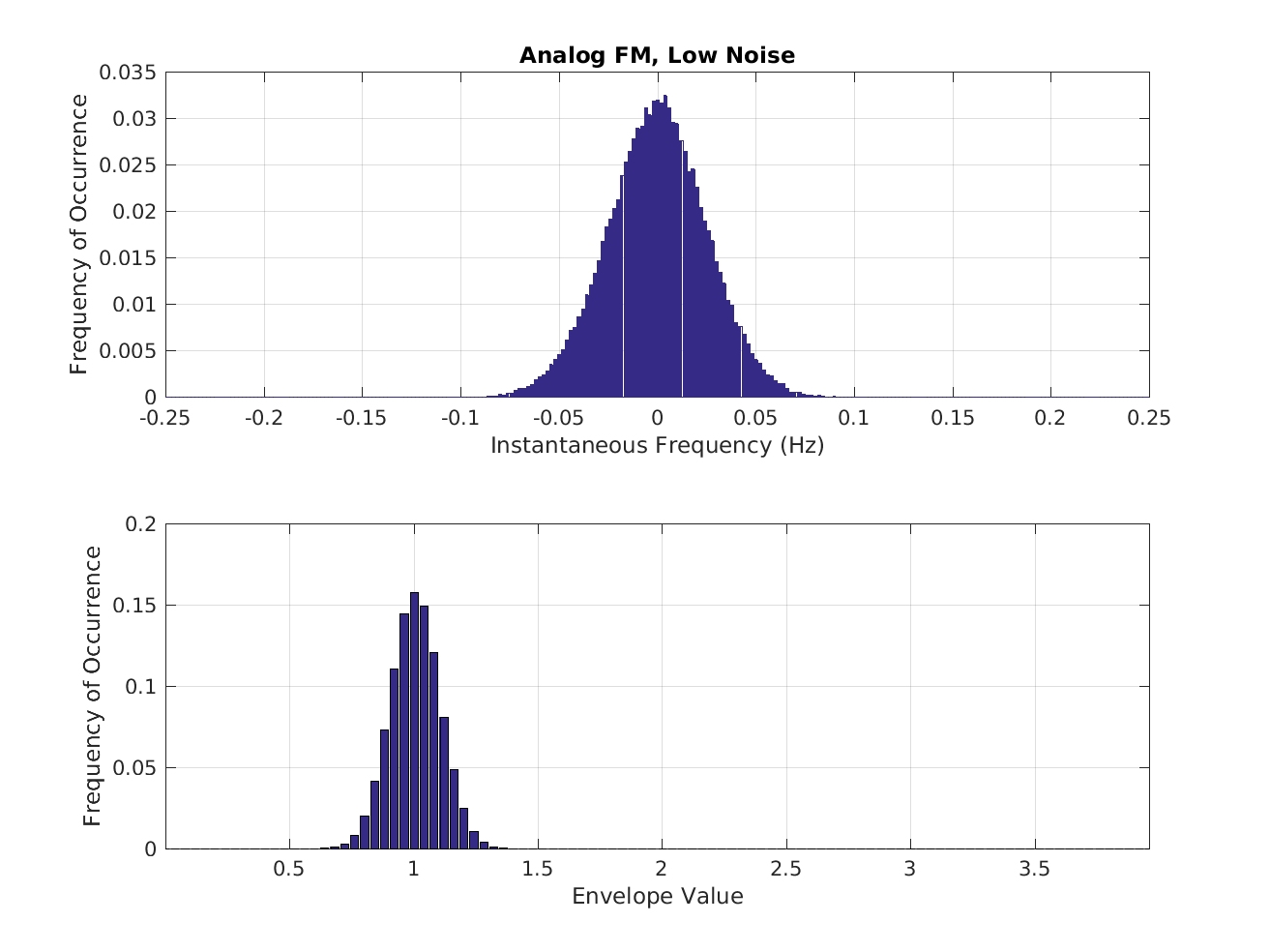As expected, the envelopes for BFSK and FM do not vary much; those for BPSK and especially AM vary quite a lot. On the other hand, the frequency content of the BFSK signal clearly shows two peaks, consistent with the signal switching randomly between two distinct frequencies as dictated by the values of the modulating bits. The frequency content of the FM signal spans a wide range compared to that for the BPSK and AM signals, which is again consistent with what we know about how the modulating waveform affects the frequency, phase, and amplitude of the sine-wave carrier.

So it is apparent that such features can be used to distinguish between these four signal types. And it isn’t hard to imagine what they might look like for other signals, such as 4FSK (four distinct peaks in the frequency histogram), OOK (two distinct peaks in the envelope histogram), etc.

Now let’s look at the same signals, but with lots of noise: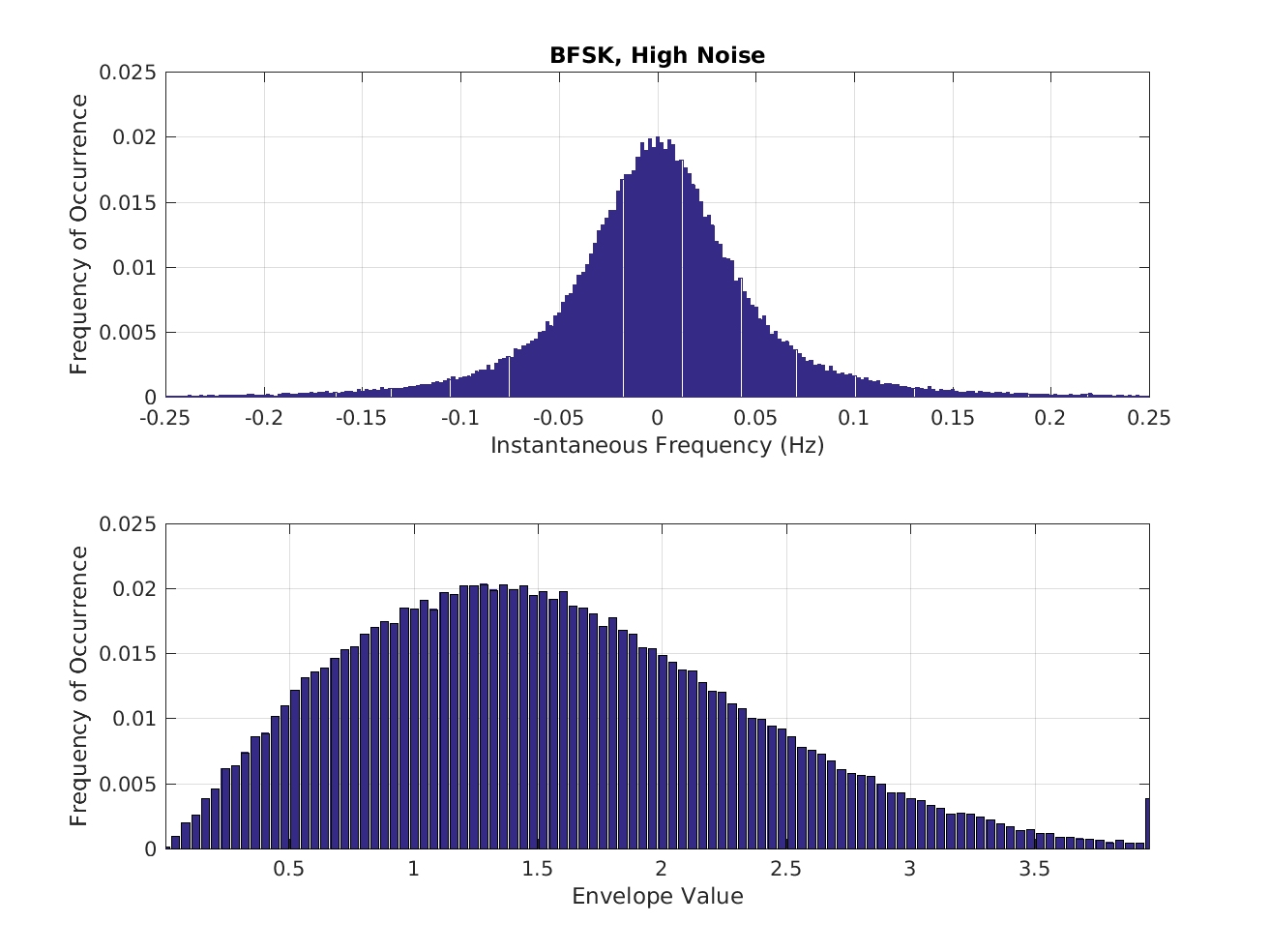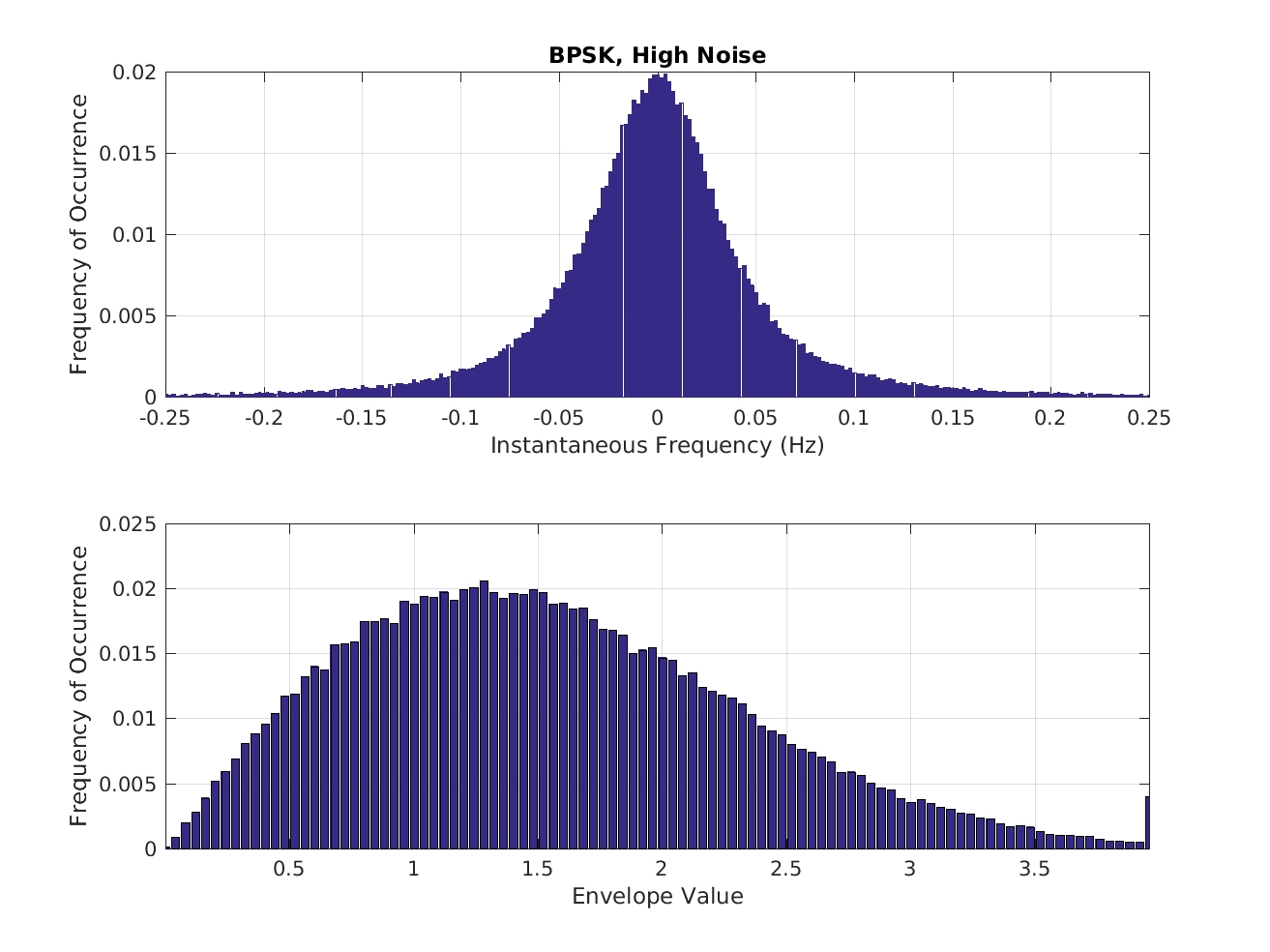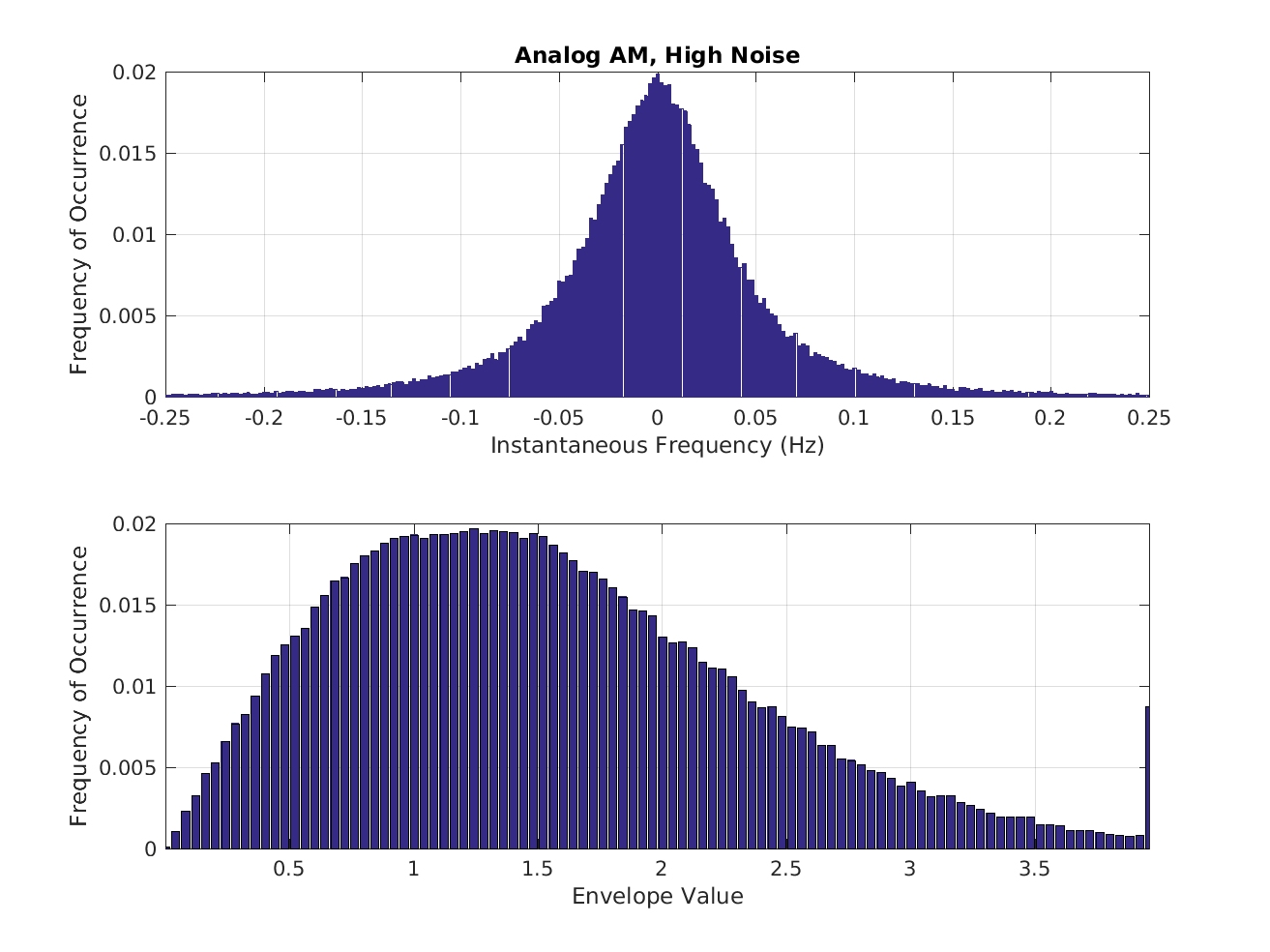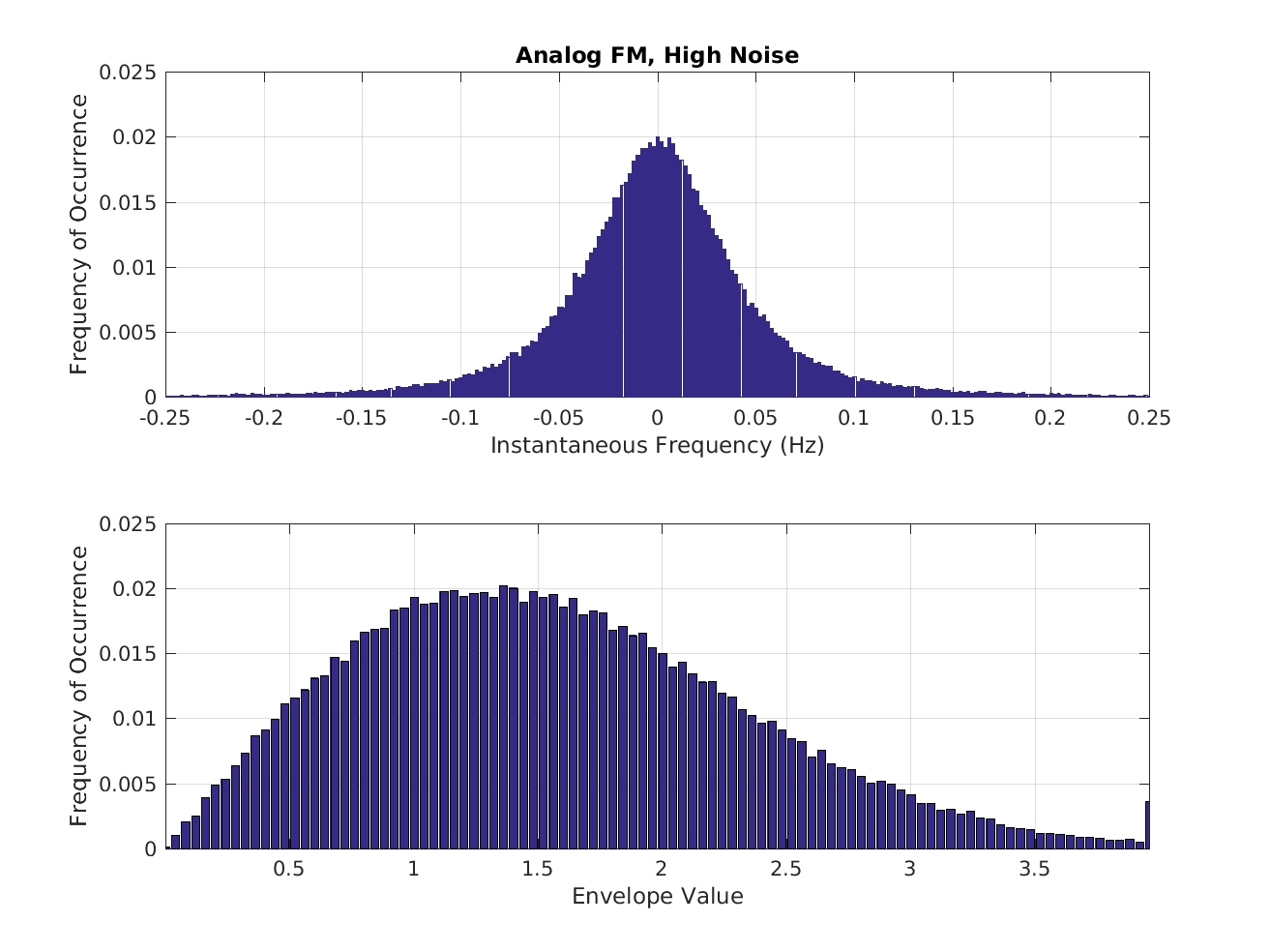Now all the features are the same. This can’t be improved by increasing the amount of data processed; that will just refine the histograms.

Finally, let’s look at the case of low noise and cochannel interference. The cochannel interferer is an MSK signal, which is a form of continuous phase modulation (CPM) and has some characteristics in common with BFSK, notably that it possesses two peaks in its own frequency histogram: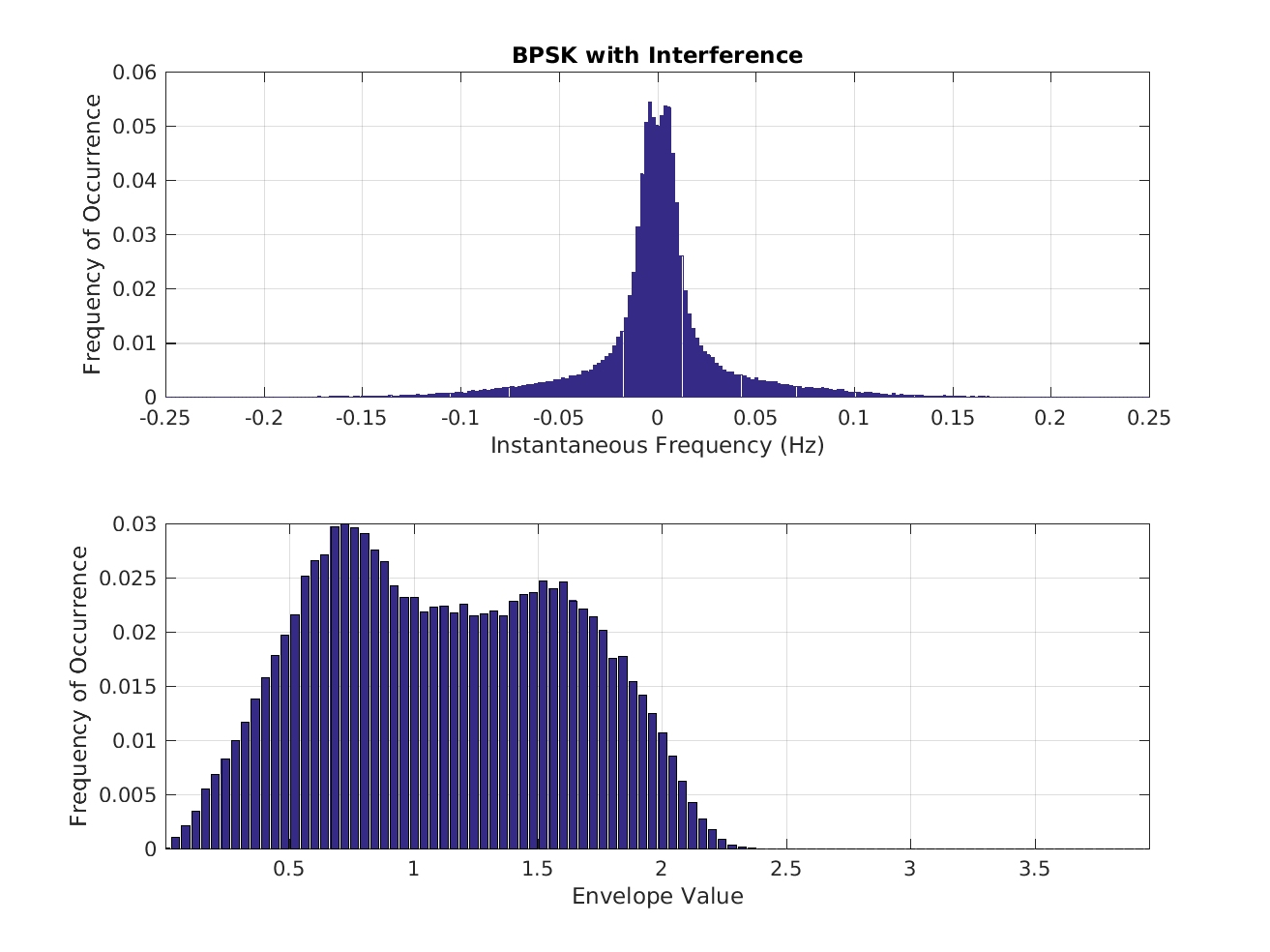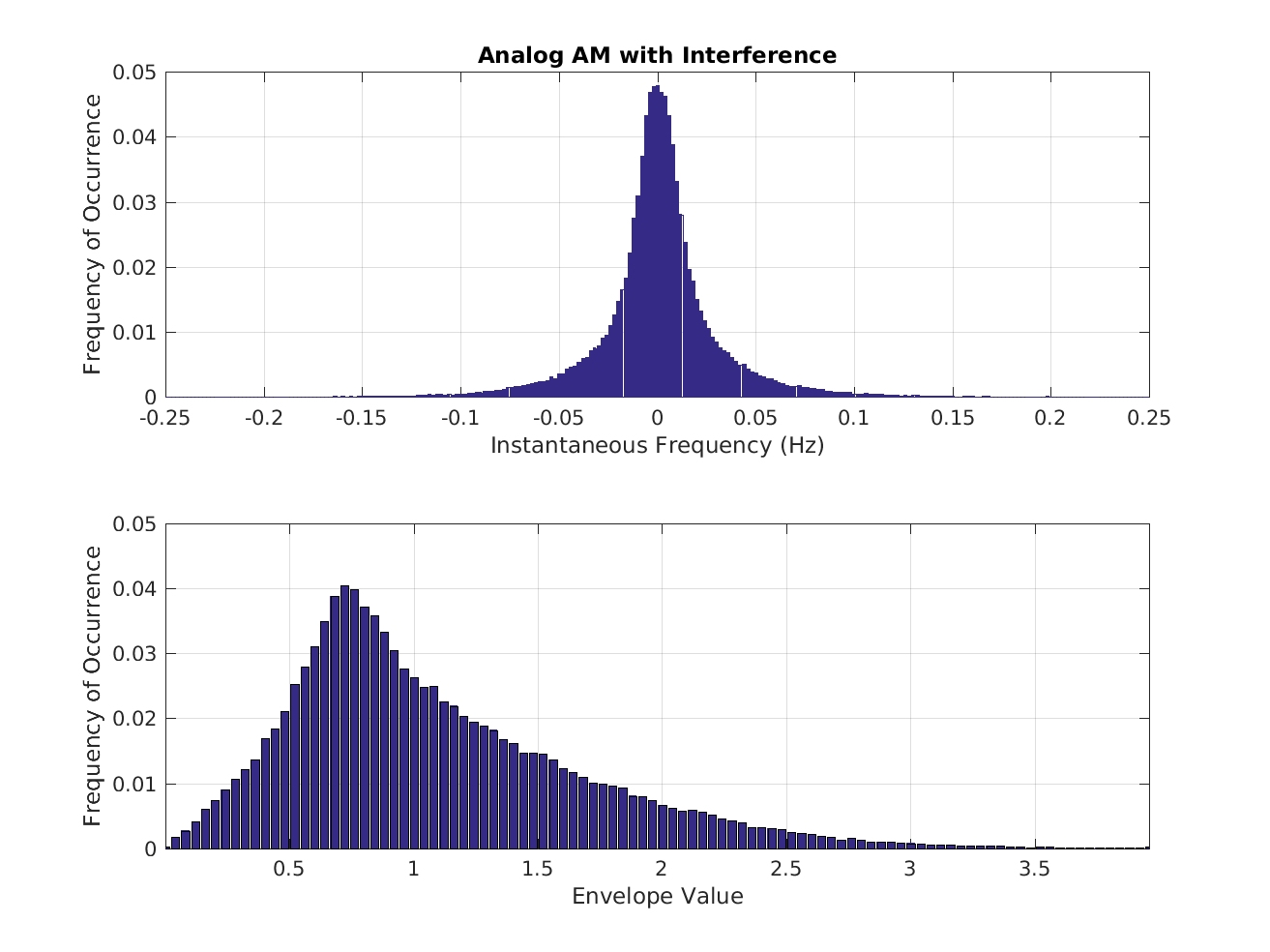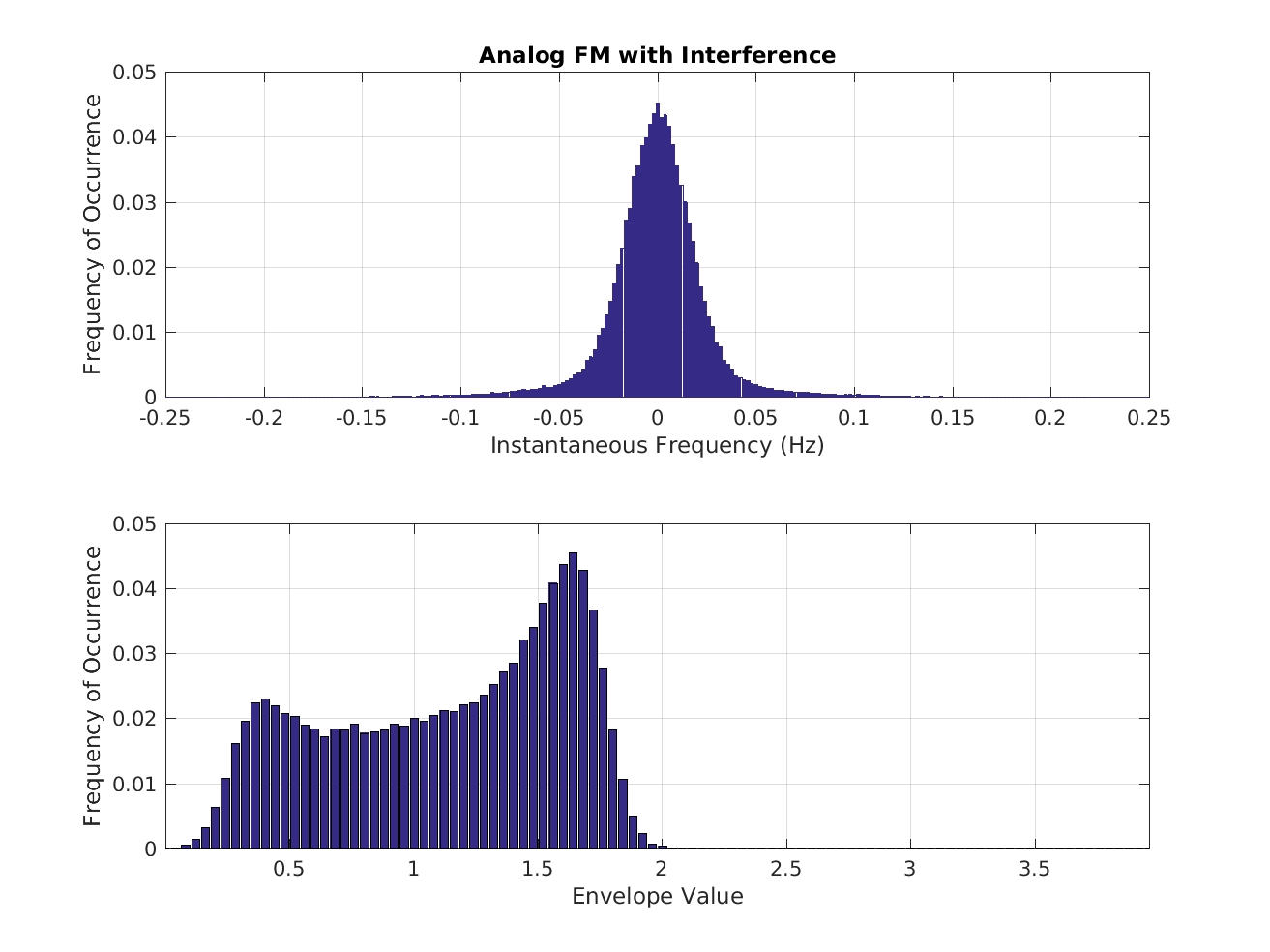So all the signals’ features are distorted and correct classification becomes difficult or impossible.

#### High-Cost Features

An example is cumulants, the estimation of which involves estimation of multiple moments, which themselves require many complex-valued multiplications and lots of additions (The Literature ). Another example is constellation-based MR, because to extract the constellation values requires good synchronization (The Literature ). Before you can attempt to blindly recognize the I/Q cluster points making up the constellation, you need to synchronize to the symbol clock and remove the residual carrier frequency. This, in turn, requires that you apply the proper matched filter for the transmitted-signal pulse type. Others have attempted to use the second-order CSP or higher-order moments of the signal or of some derived parameter (The Literature [80,82,83,84,88,89,93,94]).

And, of course, a set of high-cost features is the cyclic cumulants.

### CSP-Based Modulation Recognition Preview

So we’ve arrived at the point where we can provide motivation for the use of higher-order cyclic cumulants in modulation recognition. But exactly how should they be used?

In My Papers  I sketch an approach to deriving a cyclic-cumulant classifier. The idea is to start off by saying we have multiple hypotheses for the received data, each one of which involves the set of all possible probability density functions describing the corresponding modulation type. So, on$H_1$ we postulate that the received data has a set of probability density functions that arise from signal type 1 when it is embedded in noise. On$H_2$, the probability density functions arise from signal type 2 in noise. And then continue with as many signal types as desired.

This lends itself to  hypotheses containing multiple cochannel signals as well: the set of joint probability density functions is modified accordingly.

Then we make the claim that the set of generated hypotheses is equivalent to another set of hypotheses involving all the possible moment functions. After all, if you know all possible$n$-th order moment functions for a signal, you can reconstruct its probability density functions. This is because the series expansion for the characteristic function has coefficients equal to the moment functions, and the characteristic function is simply the Fourier transform of the probability density function.

Finally, we know that knowledge of all possible moment functions is equivalent to knowledge of all possible cumulant functions by virtue of the moment-cumulant formula. So we arrive at a set of hypotheses that involve only cumulants (and therefore, for cyclostationary signals, cyclic cumulants).  One can then derive the structure for the classifier, which essentially matches the cyclic cumulants estimated from the observed data with the ideal cyclic cumulants for each considered modulation type. We’ll elaborate on this approach in the second post on modulation recognition. In the third post I’ll provide some performance examples and I hope to post a set of signal files so others can compare their approaches (but see the posted data set for the Machine-Learner Challenge in the meantime).

For now, please leave your comments or corrections below. I’ll be adding more citations to the literature to this post over time, so if you know of a good paper on modulation recognition that I’ve not included, please also leave it in the comments.1.nisha says: